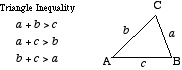index: click on a letter A B C D E F G H I J K L M N O P Q R S T U V W X Y Z A to Z index index: subject areas numbers & symbols sets, logic, proofs geometry algebra trigonometry advanced algebra & pre-calculus calculus advanced topics probability & statistics real world applications multimedia entrieswww.mathwords.com about mathwords website feedback

 Triangle Inequality A mathematical restatement of the concept that the shortest distance between two points is a straight line. The triangle inequality states that the sum of the lengths of any two sides of a triangle is greater than the length of the third side.See also Triangle inequality with absolute value